# Problem of the Month (June 2007)

Consider the equation n 12 + (n-1) 22 + (n-2) 32 + . . . + 1 n2 = k2. This equation holds for certain integer values of n and k. The first few solutions are n=1 k=1, n=6 k=14, and n=25 k=195. Can you find all the other solutions?

We can illustrate this identity for a shape S by tiling a copy of S scaled by a factor of k with: n copies of S, (n-1) copies of S scaled by a factor of 2, ... up to 1 copy of S scaled by a factor of n. These are called anti-partridge tilings. Clearly the n=1 k=1 case is trivial. Anti-partridge tilings for n=6 of squares and equilateral triangles were found a decade ago. These can be stretched to yield anti-partridge tilings for n=6 of all parallelograms and triangles. What anti-partridge tilings can you find for n=6 or n=25?

Some rare shapes can have anti-partridge tilings for other values of n. There are apparently rectangle solutions for all values of n. Can you find some? There are also solutions for n=4 and n=10 using triangles. Can you find them? What other anti-partridge tilings can you find for non-integer values of k?

Joe DeVincentis noted that n 12 + (n-1) 22 + (n-2) 32 + . . . + 1 n2 = n(n+1)2(n+2)/12, so we want n so that 3n(n+2) is a square. The n with this property are of the form [ (2 - √3)n + (2 + √3)n - 2 ] / 2.

Patrick Hamlyn claimed to find all the solutions for the 30-60-90 triangle for n=6, but George Sicherman found many more.

Here are the known non-trivial anti-partridge tilings for n=6: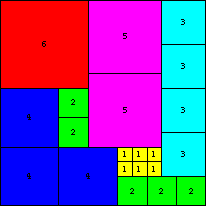(Colin Singleton, 1996)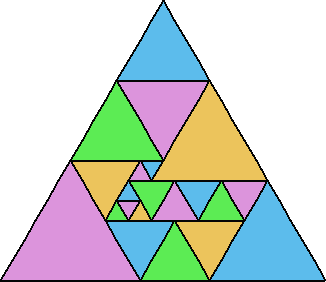(Bob Wainwright, 2000)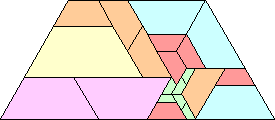(Erich Friedman, 2002)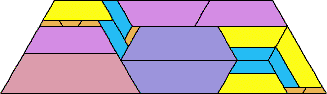(Patrick Hamlyn, 2002)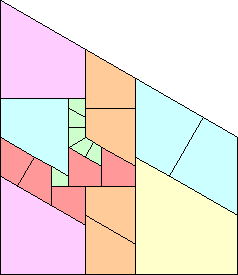(Erich Friedman, 2002)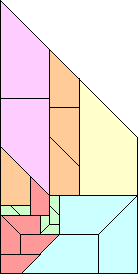(Erich Friedman, 2002)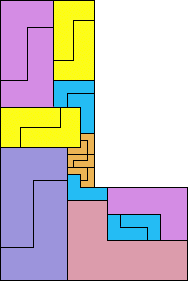(Patrick Hamlyn, 2002)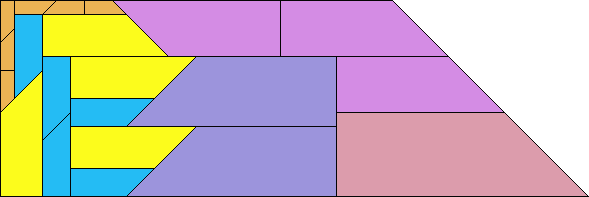(Patrick Hamlyn)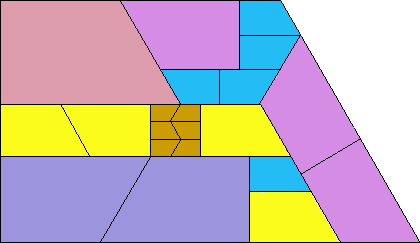(Patrick Hamlyn)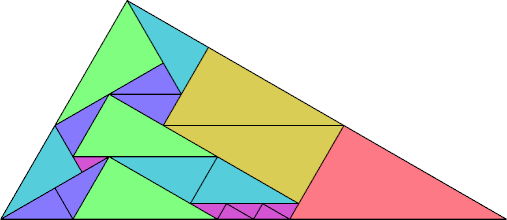(George Sicherman)
And here are the known non-trivial anti-partridge tilings for n=25:(Erich Friedman, 1998)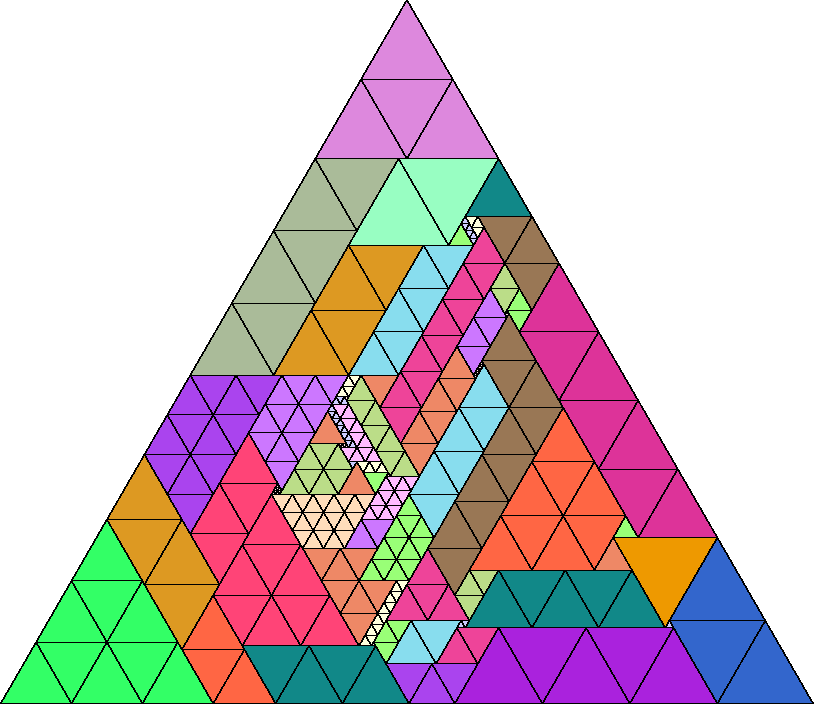(Mike Reid)

George Sicherman found many rectangles for other values of n.

Joe DeVincentis showed there are rectangle solutions for all n not equal to 1 mod 4 by stacking squares of size n+1–i above those of size i (like n=2 through 4 below).

Here are the known non-trivial anti-partridge tilings for other values of n: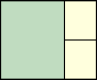n=2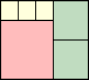n=3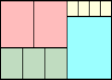n=4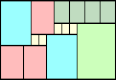n=5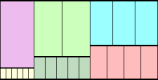n=6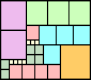n=7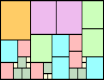n=7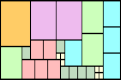n=7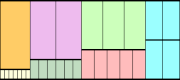n=7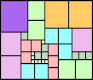n=8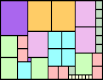n=8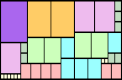n=8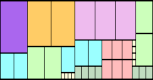n=8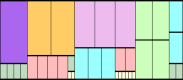n=8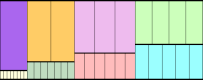n=8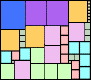n=9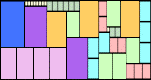n=9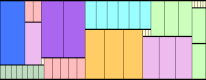n=9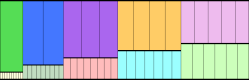n=10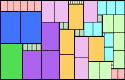n=10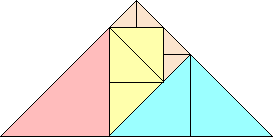n=4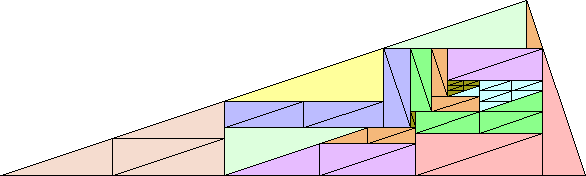n=10

Patrick Hamlyn likes tiling things on other surfaces too. He found this anti-partridge tiling for the bent triomino on a Möbius strip: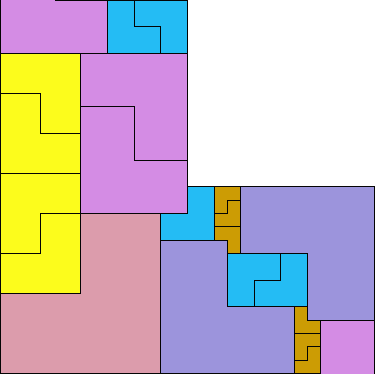Patrick Hamlyn also found anti-partridge-like tilings to illustrate the following identities:

(1) 132 + (2) 122 + . . . + (10) 42 = 552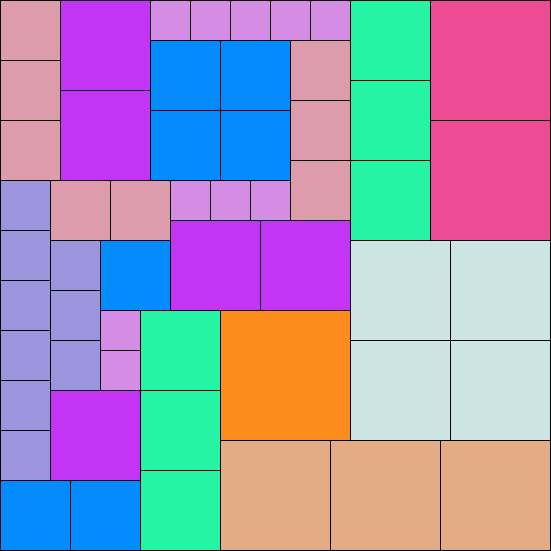(4) 102 + (5) 92 + . . . + (11) 32 = 482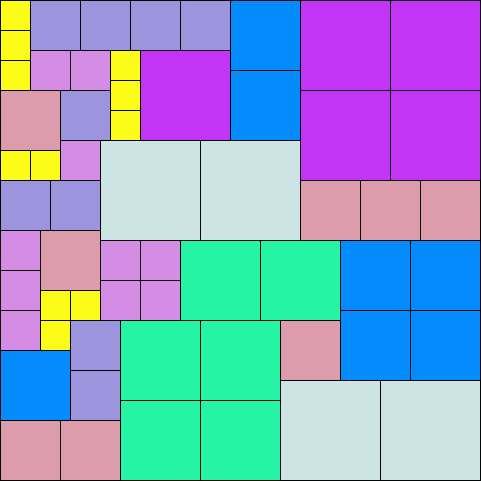(6) 72 + (7) 62 + . . . + (11) 22 = 322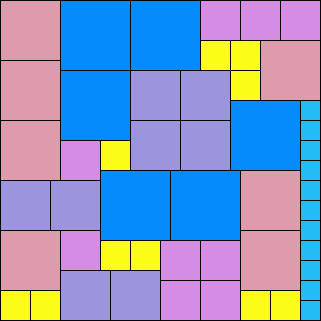(7) 82 + (8) 72 + . . . + (14) 12 = 422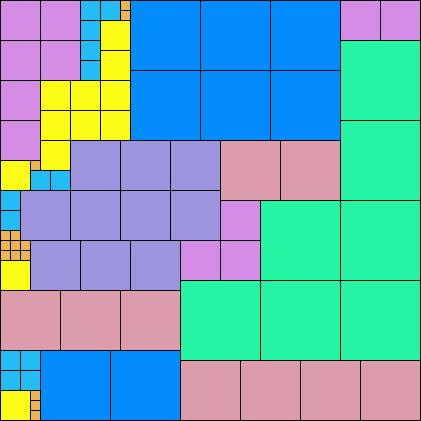Claudio Baiocchi found this anti-partridge-like tiling: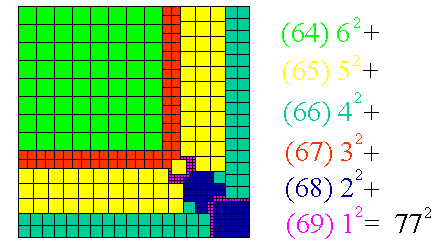If you can extend any of these results, please e-mail me. Click here to go back to Math Magic. Last updated 7/2/07.